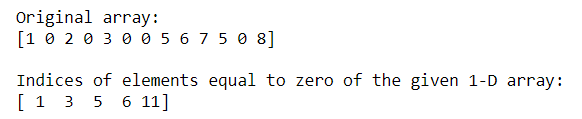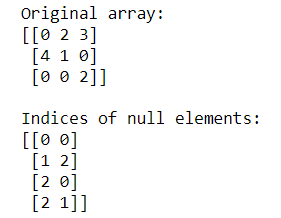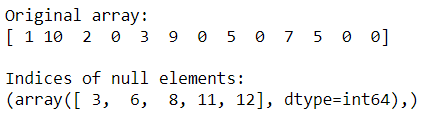# Find indices of elements equal to zero in a NumPy array

• Last Updated : 05 Sep, 2020

Sometimes we need to find out the indices of all null elements in the array. Numpy provides many functions to compute indices of all null elements.

Method 1: Finding indices of null elements using numpy.where()

This function returns the indices of elements in an input array where the given condition is satisfied.

Syntax :

```numpy.where(condition[, x, y])
When True, yield x, otherwise yield y
```

## Python3

 `# importing Numpy package``import` `numpy as np`` ` `# creating a 1-D Numpy array``n_array ``=` `np.array([``1``, ``0``, ``2``, ``0``, ``3``, ``0``, ``0``, ``5``,``                    ``6``, ``7``, ``5``, ``0``, ``8``])`` ` `print``(``"Original array:"``)``print``(n_array)`` ` `# finding indices of null elements using np.where()``print``("\nIndices of elements equal to zero of the \``given ``1``-``D array:")`` ` `res ``=` `np.where(n_array ``=``=` `0``)[``0``]``print``(res)`

Output:Method 2: Finding indices of null elements using numpy.argwhere()

This function is used to find the indices of array elements that are non-zero, grouped by element.

Syntax

```numpy.argwhere(arr)
```

## Python3

 `# importing Numpy package``import` `numpy as np`` ` `# creating a 3-D Numpy array``n_array ``=` `np.array([[``0``, ``2``, ``3``],``                    ``[``4``, ``1``, ``0``],``                    ``[``0``, ``0``, ``2``]])`` ` `print``(``"Original array:"``)``print``(n_array)`` ` `# finding indices of null elements ``# using np.argwhere()``print``(``"\nIndices of null elements:"``)``res ``=` `np.argwhere(n_array ``=``=` `0``)`` ` `print``(res)`

Output:Method 3: Finding the indices of null elements using numpy.nonzero()

This function is used to Compute the indices of the elements that are non-zero. It returns a tuple of arrays, one for each dimension of arr, containing the indices of the non-zero elements in that dimension.

Syntax:

`numpy.nonzero(arr)`

## Python3

 `# importing Numpy package``import` `numpy as np`` ` `# creating a 1-D Numpy array``n_array ``=` `np.array([``1``, ``10``, ``2``, ``0``, ``3``, ``9``, ``0``, ``                    ``5``, ``0``, ``7``, ``5``, ``0``, ``0``])`` ` `print``(``"Original array:"``)``print``(n_array)`` ` `# finding indices of null elements using ``# np.nonzero()``print``(``"\nIndices of null elements:"``)`` ` `res ``=` `np.nonzero(n_array ``=``=` `0``)``print``(res)`

Output:My Personal Notes arrow_drop_up